# The OPTMILP Procedure

### Example 11.1 Simple Integer Linear Program

This example illustrates a model in an MPS-format SAS data set. This data set is passed to PROC OPTMILP, and a solution is found.

Consider a scenario where you have a container with a set of limiting attributes (volume V and weight W) and a set I of items that you want to pack. Each item type i has a certain value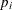, a volume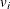, and a weight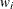. You must choose at most four items of each type so that the total value is maximized and all the chosen items fit into the container. Letbe the number of items of type i to be included in the container. This model can be formulated as the following integer linear program: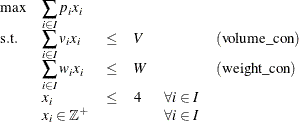Constraint (volume_con) enforces the volume capacity limit, while constraint (weight_con) enforces the weight capacity limit. An instance of this problem can be saved in an MPS-format SAS data set by using the following code:

data ex1data;
input field1 $field2$ field3 $field4 field5$ field6;
datalines;
NAME       .             ex1data           .     .                 .
ROWS       .             .                 .     .                 .
MAX        z             .                 .     .                 .
L          volume_con    .                 .     .                 .
L          weight_con    .                 .     .                 .
COLUMNS    .             .                 .     .                 .
.          .MRK0         'MARKER'          .     'INTORG'          .
.          x          z                 1     volume_con       10
.          x          weight_con       12     .                 .
.          x          z                 2     volume_con      300
.          x          weight_con       15     .                 .
.          x          z                 3     volume_con      250
.          x          weight_con       72     .                 .
.          x          z                 4     volume_con      610
.          x          weight_con      100     .                 .
.          x          z                 5     volume_con      500
.          x          weight_con      223     .                 .
.          x          z                 6     volume_con      120
.          x          weight_con       16     .                 .
.          x          z                 7     volume_con       45
.          x          weight_con       73     .                 .
.          x          z                 8     volume_con      100
.          x          weight_con       12     .                 .
.          x          z                 9     volume_con      200
.          x          weight_con      200     .                 .
.          x         z                10     volume_con       61
.          x         weight_con      110     .                 .
.          .MRK1         'MARKER'          .     'INTEND'          .
RHS        .             .                 .     .                 .
.          .RHS.         volume_con     1000     .                 .
.          .RHS.         weight_con      500     .                 .
BOUNDS     .             .                 .     .                 .
UP         .BOUNDS.      x              4     .                 .
UP         .BOUNDS.      x              4     .                 .
UP         .BOUNDS.      x              4     .                 .
UP         .BOUNDS.      x              4     .                 .
UP         .BOUNDS.      x              4     .                 .
UP         .BOUNDS.      x              4     .                 .
UP         .BOUNDS.      x              4     .                 .
UP         .BOUNDS.      x              4     .                 .
UP         .BOUNDS.      x              4     .                 .
UP         .BOUNDS.      x             4     .                 .
ENDATA     .             .                 .     .                 .
;


In the COLUMNS section of this data set, the name of the objective is z, and the objective coefficientsappear in field4. The coefficientsof (volume_con) appear in field6. The coefficientsof (weight_con) appear in field4. In the RHS section, the bounds V and W appear in field4. This problem can be solved by using the following statements to call the OPTMILP procedure:


proc optmodel;
num nItems          = 10;
num volume_capacity = 1000;
num weight_capacity = 500;
set<num> Items = {1..nItems};
num value{Items}  = [1,2,3,4,5,6,7,8,9,10];
num volume{Items} = [10, 300, 250, 610, 500, 120, 45, 100, 200, 61 ];
num weight{Items} = [12,  15,  72, 100, 223,  16, 73,  12, 200, 110];
var x{Items} integer >= 0 <= 4;
max z = sum{i in Items} value[i] * x[i];
con volume_con: sum{i in Items} volume[i] * x[i] <= volume_capacity;
con weight_con: sum{i in Items} weight[i] * x[i] <= weight_capacity;
save mps ex1data;
quit;
run;
proc optmilp data=ex1data primalout=ex1soln;
run;


The progress of the solver is shown in Output 11.1.1.

Output 11.1.1: Simple Integer Linear Program PROC OPTMILP Log

 NOTE: The problem ex1data has 10 variables (0 binary, 10 integer, 0 free, 0 fixed). NOTE: The problem has 2 constraints (2 LE, 0 EQ, 0 GE, 0 range). NOTE: The problem has 20 constraint coefficients. NOTE: The MILP presolver value AUTOMATIC is applied. NOTE: The MILP presolver removed 0 variables and 0 constraints. NOTE: The MILP presolver removed 0 constraint coefficients. NOTE: The MILP presolver modified 0 constraint coefficients. NOTE: The presolved problem has 10 variables, 2 constraints, and 20 constraint coefficients. NOTE: The MILP solver is called. Node  Active    Sols    BestInteger      BestBound      Gap    Time 0       1       3     85.0000000    178.0000000   52.25%       0 0       1       3     85.0000000     88.0955497    3.51%       0 0       1       3     85.0000000     88.0626822    3.48%       0 0       1       3     85.0000000     87.9666563    3.37%       0 0       1       3     85.0000000     87.9661593    3.37%       0 0       1       3     85.0000000     87.8181818    3.21%       0 NOTE: The MILP solver added 2 cuts with 13 cut coefficients at the root. 3       0       5     87.0000000     87.0000000    0.00%       0 NOTE: Optimal. NOTE: Objective = 87. NOTE: The data set WORK.EX1SOLN has 10 observations and 8 variables.

The data set ex1soln is shown in Output 11.1.2.

Output 11.1.2: Simple Integer Linear Program Solution

 Example 1 Solution Data

Objective
Function ID
RHS ID Variable Name Variable
Type
Objective
Coefficient
Lower
Bound
Upper
Bound
Variable
Value
z .RHS. x I 1 0 4 0
z .RHS. x I 2 0 4 0
z .RHS. x I 3 0 4 0
z .RHS. x I 4 0 4 0
z .RHS. x I 5 0 4 0
z .RHS. x I 6 0 4 3
z .RHS. x I 7 0 4 1
z .RHS. x I 8 0 4 4
z .RHS. x I 9 0 4 0
z .RHS. x I 10 0 4 3

The optimal solution is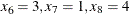, and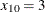, with a total value of 87. From this solution, you can compute the total volume used, which is 988 (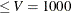); the total weight used is 499 (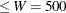). The problem summary and solution summary are shown in Output 11.1.3.


proc optmodel;
num nItems          = 10;
num volume_capacity = 1000;
num weight_capacity = 500;
set<num> Items = {1..nItems};
num value{Items}  = [1,2,3,4,5,6,7,8,9,10];
num volume{Items} = [10, 300, 250, 610, 500, 120, 45, 100, 200, 61 ];
num weight{Items} = [12,  15,  72, 100, 223,  16, 73,  12, 200, 110];
var x{Items} integer >= 0 <= 4;
max z = sum{i in Items} value[i] * x[i];
con volume_con: sum{i in Items} volume[i] * x[i] <= volume_capacity;
con weight_con: sum{i in Items} weight[i] * x[i] <= weight_capacity;
save mps ex1data;
quit;
run;
proc optmilp data=ex1data primalout=ex1soln;
title ' ';
run;


Output 11.1.3: Simple Integer Linear Program Summary

The OPTMILP Procedure

Problem Summary
Problem Name ex1data
Objective Sense Maximization
Objective Function z
RHS .RHS.

Number of Variables 10
Bounded Above 0
Bounded Below 0
Bounded Above and Below 10
Free 0
Fixed 0
Binary 0
Integer 10

Number of Constraints 2
LE (<=) 2
EQ (=) 0
GE (>=) 0
Range 0

Constraint Coefficients 20

Performance Information
Execution Mode Single-Machine
Number of Threads 4

Solution Summary
Solver MILP
Algorithm Branch and Cut
Objective Function z
Solution Status Optimal
Objective Value 87

Relative Gap 0
Absolute Gap 0
Primal Infeasibility 0
Bound Infeasibility 0
Integer Infeasibility 0

Best Bound 87
Nodes 4
Iterations 31
Presolve Time 0.00
Solution Time 0.00